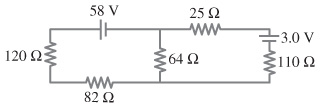# Problem: Calculate the currents in the below resistors of the figure.Part ACalculate the current in 25 ohmresistor.Express your answer to two significant figures and include the appropriate unitsPart BCalculate the current in 120 ohm resistor.Express your answer to two significant figures and include the appropriate units.

###### FREE Expert Solution
96% (48 ratings)View Complete Written Solution
###### Problem DetailsCalculate the currents in the below resistors of the figure.

Part A

Calculate the current in 25 ohmresistor.

Express your answer to two significant figures and include the appropriate units

Part B

Calculate the current in 120 ohm resistor.

Express your answer to two significant figures and include the appropriate units.